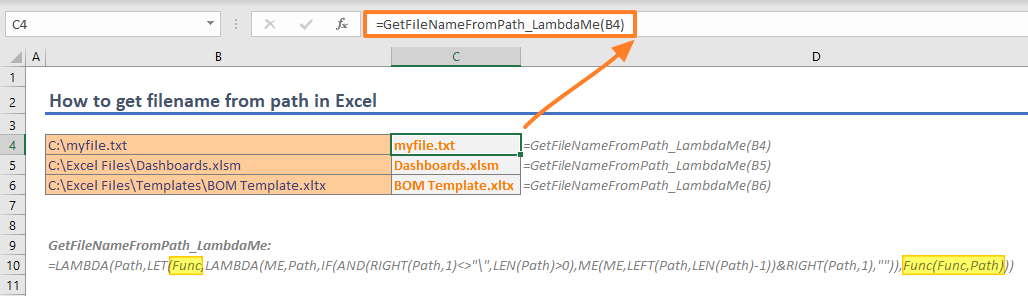In this guide, we’re going to show you how to get filename from path in Excel. We will cover how to do this with and without VBA.

## File path and file name

A file path is a string identifier that specifies the unique location in a file system. It contains folders in a hierarchical order following by a file name. Each element is separated by a delimiter which is usually a backslash "\". The goal of getting filename from path is to parse that filename after the last delimiter.Warning: If your paths contain another separator, update the formulas by replacing backslash ("\") with the separator character fits your case.

We will show you four different approaches to get filename from path in Excel.

## Conservative method

Our first approach is using well known Excel functions MIN, SUBSTITUE and LEN to get the file name. You can use this formula in any Excel version.

=MID(<path>,FIND("*",SUBSTITUTE(<path>,"\","*",LEN(<path>)-LEN(SUBSTITUTE(<path>,"\",""))))+1,LEN(<path>))

This formula has couple of steps:

1. At the inner section, all separators ("\") get replaced with empty strings.
2. The formula subtracts the length of substituted path (1) from original path to find the number of separators.
3. The outer SUBSTITUTE function replaces the last separator ("\") with "*". Obviously, the last separator's instance is equal to number of separators (2).
4. Finally, the FIND locates the "*" character, and the MID function parses the file name after the character.## VBA with FileSystemObject

You can use VBA to create your own custom functions which you can use in worksheet as well. Of course, you can use this function in your macros as well.

The code is very short. It uses GetFileName method of FileSystemObject object. The important section of the code is the initializing of the object. The following code sets FileSystemObject object to fso variable.

Set fso = CreateObject("Scripting.FileSystemObject")

Once the object is initialized, use the GetFileName method by providing the path.

GetFileNameFromPath_FSO = fso.GetFileName("C:\Excel Files\Dashboards.xlsm")

The above line returns "Dashboard.xlsm" string. Here is the function version you can use in your worksheets as well.

```Function GetFileNameFromPath_FSO(ByVal Path As String) As String
Set fso = CreateObject("Scripting.FileSystemObject")
GetFileNameFromPath_FSO = fso.GetFileName(Path)
End Function```## VBA with a recursive function

A recursive function is function which calls itself. The recursive approach acts like an iteration and helps us to parse values starting from the end of a string. You could have used recursive functions only in VBA until the LAMBDA function has been released. Because the most of Excel users do not have access to the LAMBDA function, we will show you VBA version which any Excel user can use.

This function has only few rows as well. The function's name is GetFilenameFromPath_Recursive and take a single argument named Path.

The first row is a logical test that checks if the last character in the argument is a backslash ("\") or not and if the argument is not an empty string. If the test is passed, the function returns itself with the argument without its last character and the last character of the path. This is where the recursion occurs.

The function runs itself until coming up a backslash ("\") or not a character left. It parses the characters from the right side and combines them with each run.

The last row contains a standard End If statement which determines where the If block ends.

```Function GetFilenameFromPath_Recursive(ByVal Path As String) As String
If Right\$(Path, 1) <> "\" And Len(Path) > 0 Then
GetFilenameFromPath_Recursive = GetFilenameFromPath_Recursive(Left\$(Path, Len(Path) - 1)) & Right\$(Path, 1)
End If
End Function```## Using LAMBDA to get filename from path

If you are Microsoft 365 subscriber, you can create recursive functions without using VBA. Briefly, the LAMBDA function is a special function that converts named ranges into user defined functions. Its syntax allows you to define arguments and a custom formula which uses that defined arguments.

For example, let's say my custom function will have two arguments and returns multiplication of two arguments. All I need to is creating a named range, such as "MyLambda" and enter the following formula into Refers to box.

 Syntax Sample Formula Sample Result =LAMBDA(x, y, x*y) =MyLambda(2,3) 6If you call the named range "MyLambda" in the "MyLambda" function, you will create a recursive function. Same logic can be applied to VBA function at previous section.

The following is the LAMBDA version of our VBA code. The function's name is GetFileNameFromPath_Lambda. Check out how the function calls itself after IF function's logical test.

=LAMBDA(Path,IF(AND(RIGHT(Path,1)<>"\",LEN(Path)>0),GetFileNameFromPath_Lambda(LEFT(Path,LEN(Path)-1))&RIGHT(Path,1),""))Warning: Do not forget to update formula name in the formula if you change the named range's name. Otherwise, the function returns #NAME? error due to incorrect function name.

## Using LAMBDA Function with different approach

Alternatively, you can use the LAMBDA function without calling the "function name".  The definition may sound complicated since you must call the function in the function by its name. This structure dictates you to update each occurrence of the name every time change the function's name.

You can overcome this necessity by using another Microsoft 365-specific function called LET. The LET function allows you to define named ranges in a formula scope. You can define repeating values or blocks into these names and use them continuously.

If you define the name of the LAMBDA function in the formula, you can use the in-formula name over and over to make the function recursive. In our example, we create the name "Func" in the formula and call it within self. Outer LAMBDA function is to give the whatever name we want, "GetFileNameFromPath_LambdaMe".

=LAMBDA(Path,LET(Func,LAMBDA(ME,Path,IF(AND(RIGHT(Path,1)<>"\",LEN(Path)>0),ME(ME,LEFT(Path,LEN(Path)-1))&RIGHT(Path,1),"")),Func(Func,Path)))